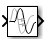# Transport Delay

Delay input by given amount of time

• Library:## Description

The Transport Delay block delays the input by a specified amount of time. You can use this block to simulate a time delay. The input to this block should be a continuous signal.

At the start of simulation, the block outputs the Initial output parameter until the simulation time exceeds the Time delay parameter. Then, the block begins generating the delayed input. During simulation, the block stores input points and simulation times in a buffer. You specify this size with the Initial buffer size parameter.

When you want output at a time that does not correspond to times of the stored input values, the block interpolates linearly between points. When the delay is smaller than the step size, the block extrapolates from the last output point, which can produce inaccurate results. Because the block does not have direct feedthrough, it cannot use the current input to calculate an output value. For example, consider a fixed-step simulation with a step size of 1 and the current time at t = 5. If the delay is 0.5, the block must generate a point at t = 4.5. Because the most recent stored time value is at t = 4, the block performs forward extrapolation.

The Transport Delay block does not interpolate discrete signals. Instead, the block returns the discrete value at the required time.

This block differs from the Unit Delay block, which delays and holds the output on sample hits only.

### Tip

Avoid using `linmod` to linearize a model that contains a Transport Delay block. For more information, see Linearizing Models.

## Ports

### Input

expand all

Input signal to delay, specified as a scalar, vector, or matrix.

Data Types: `double`

### Output

expand all

Input signal, delayed by specified amount of time. Output has the same dimensions and data type as the input signal.

Data Types: `double`

## Parameters

expand all

Specify the amount of simulation time to delay the input signal before propagation to the output as a nonnegative scalar, vector, or matrix.

#### Programmatic Use:

 Block Parameter: `DelayTime` Type: character vector, string Values: nonnegative scalar, vector, or matrix Default: `'1'`

Specify the output that the block generates until the simulation time first exceeds the time delay input as a scalar, vector, or matrix.

#### Limitations

The initial output of this block cannot be `inf` or `NaN`.

A `Run-to-run tunable parameter` cannot be changed during a simulation run time. However, changing it before a simulation begins does not cause Accelerator or Rapid Accelerator to regenerate code.

#### Programmatic Use

 Block Parameter: `InitialOutput` Type: character vector, string Values: scalar | vector | matrix Default: `'0'`

Define the initial memory allocation for the number of input points to store.

• If the number of input points exceeds the initial buffer size, the block allocates additional memory.

• After simulation ends, a message shows the total buffer size needed.

#### Tips

• Because allocating memory slows down simulation, choose this value carefully if simulation speed is an issue.

• For long time delays, this block can use a large amount of memory, particularly for dimensionalized input.

#### Programmatic Use

 Block Parameter: `BufferSize` Type: character vector, string Value: positive integer scalar Default: `'1024'`

Select this check box to use a fixed-size buffer to save input data from previous time steps.

The Initial buffer size parameter specifies the size of the buffer. If the buffer is full, new data replaces data already in the buffer. Simulink® software uses linear extrapolation to estimate output values that are not in the buffer.

### Note

If you have a Simulink Coder™ license, ERT or GRT code generation uses a fixed-size buffer even if you do not select this check box.

#### Tips

• If the input data is linear, selecting this check box can save memory.

• If the input data is nonlinear, do not select this check box. Doing so can yield inaccurate results.

#### Programmatic Use

 Block Parameter: `FixedBuffer` Type: character vector, string Value: `'off'` | `'on'` Default: `'off'`

Cause the block to output its input during linearization and trim, which sets the block mode to direct feedthrough.

#### Tips

• Selecting this check box can cause a change in the ordering of states in the model when you use the functions `linmod`, `dlinmod`, or `trim`. To extract this new state ordering:

1. Compile the model using the following command, where `model` is the name of the Simulink model.

` [sizes, x0, x_str] = model([],[],[],'lincompile'); `
2. Terminate the compilation with the following command.

` model([],[],[],'term'); `
• The output argument `x_str`, which is a cell array of the states in the Simulink model, contains the new state ordering. When you pass a vector of states as input to the `linmod`, `dlinmod`, or `trim` functions, the state vector must use this new state ordering.

#### Programmatic Use

 Block Parameter: `TransDelayFeedthrough` Type: character vector, string Value: `'off'` | `'on'` Default: `'off'`

Set the order of the Pade approximation for linearization routines as a scalar, vector, or matrix of nonnegative integers.

• The default value is `0`, which results in a unity gain with no dynamic states.

• Setting the order to a positive integer `n` adds `n` states to your model, but results in a more accurate linear model of the transport delay.

#### Programmatic Use

 Block Parameter: `PadeOrder` Type: character vector, string Values: scalar | vector | matrix Default: `'0'`

## Block Characteristics

 Data Types `double` Direct Feedthrough `yes` Multidimensional Signals `no` Variable-Size Signals `no` Zero-Crossing Detection `no`